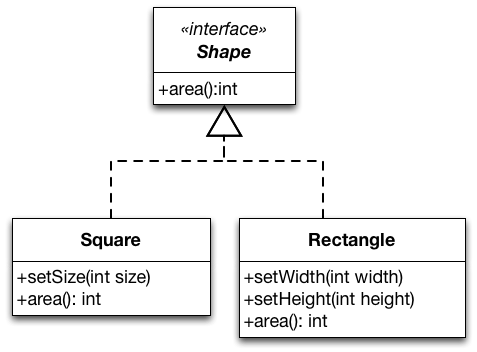# Liskov substitution principle

##### Principle

When extending a class, consider that you should be able to pass objects of the subclass in place of objects of the parent class without breaking the client code.

I've stated this principle informally; if you look it up on Wikipedia for instance, you will find formal descriptions such as

##### caution

Let `ϕ(x)` be a property provable about objects `x` of type `T`. Then `ϕ(y)` should be true for objects `y` of type `S` where `S` is a sub-type of `T`.

All this, is a fancy way of saying that every subclass/derived class should be substitutable for their base/parent class. In other words, a subclass should not break the expectations (code contracts) set by its super-class.1

Let's see an example to better understand the Liskov Substitution Principle (LSP). Consider the following implementation of `Rectangle` class.

public class Rectangle {
private int width;
private int height;
public void setWidth(int width) { this.width = width; }
public void setHeight(int height) { this.height = height; }
public int getArea() { return width*height; }
}

Since a square is a rectangle (mathematically speaking), we decide to implement `Square` as a subclass of `Rectangle`. We override `setWidth` and `setHeight` and we can reuse the implementation of `getArea`.

public class Square extends Rectangle {
@Override
public void setWidth(int width) {
super.setWidth(width);
super.setHeight(width);
}
@Override
public void setHeight(int height) {
super.setWidth(height);
super.setHeight(height);
}
}
##### tip

With this overriding of `setWidth` and `setHeight` -- to set both dimensions to the same value -- instances of `Square` remain mathematically valid squares.

The bad news is that making `Square` a subtype of `Rectangle` violates the Liskov Substitution Principle. Here is why:

void clientMethod(Rectangle rect) {
rect.setWidth(5);
rect.setHeight(4);
assert rect.getArea() == 20;
}

LSP is violated because if we pass an object of `Square` to `clientMethod` (which we can since `Square` is a subtype of `Rectangle`) the assertion in `clientMethod` will fail. In other words, the expected behavior of super type is not maintained.

So what is the solution? Well, you can simply make two separate classes: `Rectangle` and `Square`.You could use composition to reuse the code in `Rectangle`:

public class Square {
private Rectangle rectangle;
public void setSideLength(int side) {
rectangle.setHeight(side);
rectangle.setWidth(side);
}
public int getArea() { return rectangle.getArea(); }
}
##### Case in point
• LSP is all about well-designed inheritance. If you cannot substitute your baseclass with a subclass without things going wrong, then it means you used inheritance incorrectly.
• Inheritance seems like a cool idea; you get to create a hierarchy of types and reuse your code. But, it’s easy to abuse inheritance. So, don't overdo it; especially consider composition over inheritance!

1. A closely related principle in software development is called "Design by contract".